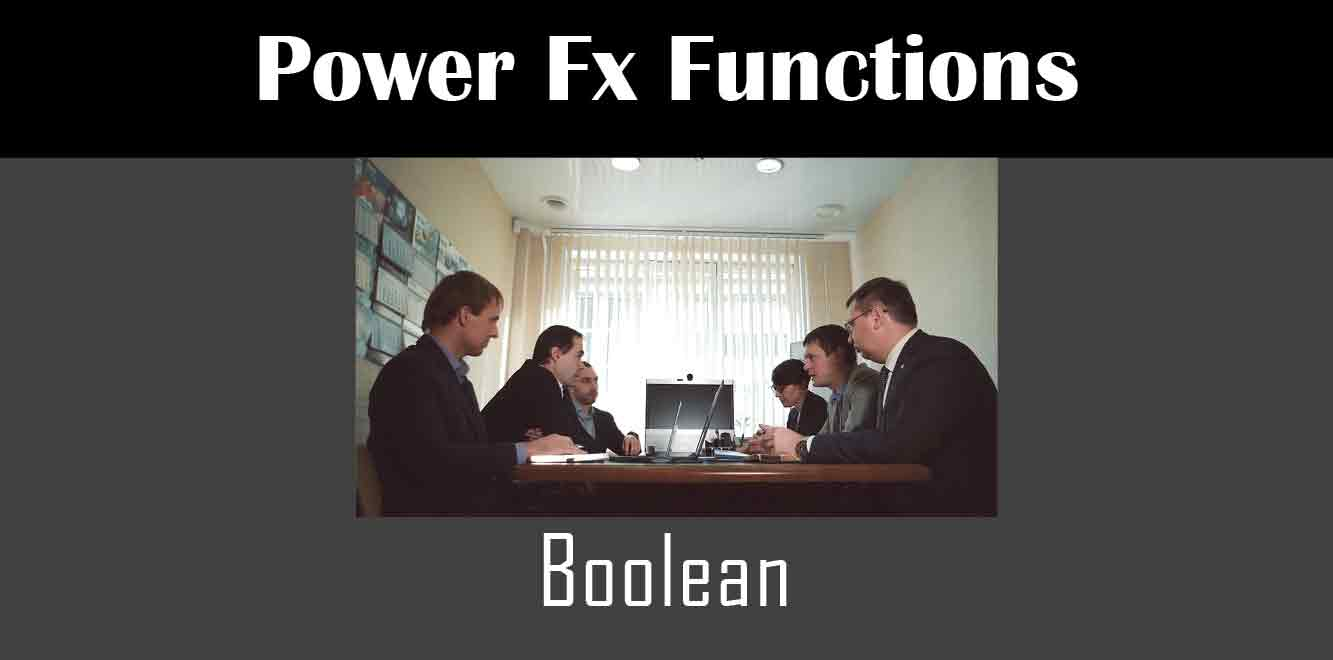# Boolean

## Y## What is the Power Fx Boolean Function?

The Power Fx Boolean function is used to evaluate True or False conditions in Power Apps. It is a logical function that returns a Boolean value of either True or False based on the condition that is specified. The syntax for the Power Fx Boolean function is as follows:

`Boolean( condition )`

The condition can be any expression that evaluates to either True or False. The Boolean function will return True if the condition evaluates to True, and False if the condition evaluates to False.

## Understanding True and False Conditions

Before we dive into how to use the Power Fx Boolean function, let’s first understand what True and False conditions are. In Power Apps, a True condition is one that evaluates to True, while a False condition is one that evaluates to False.

For example, if you have a condition that checks whether a certain value is greater than 10, and the value is indeed greater than 10, then the condition would evaluate to True. On the other hand, if the value is less than or equal to 10, the condition would evaluate to False.

## Using the Power Fx Boolean Function in Power Apps

Now that we understand what True and False conditions are, let’s discuss how to use the Power Fx Boolean function in Power Apps. Here are some examples of how to use the function:

### Example 1: Checking if a Value is True

Suppose you have a checkbox control in your Power App that a user can check or uncheck. You can use the Power Fx Boolean function to determine whether the checkbox is checked or not. Here is an example:

``` If( Boolean( Checkbox1.Value ), "Checkbox is checked", "Checkbox is not checked" ) ```

In this example, the Boolean function is used to check whether the value of the Checkbox1 control is True or False. If the value is True (i.e., the checkbox is checked), the function will return the text “Checkbox is checked”. If the value is False (i.e., the checkbox is not checked), the function will return the text “Checkbox is not checked”.

### Example 2: Checking if a Value is False

Suppose you have a dropdown control in your Power App that allows a user to select a value. You can use the Power Fx Boolean function to determine if the user has selected a specific value or not. Here is an example:

``` If( Not Boolean( IsBlank( Dropdown1.Selected.Value ) ), "Value is selected", "Value is not selected" ) ```

In this example, the Boolean function is used to check whether the value of the Dropdown1 control is blank or not. If the value is not blank (i.e., a value is selected), the function will return the text “Value is selected”. If the value is blank (i.e., no value is selected), the function will return the text “Value is not selected”.

### Example 3: Using the Boolean Function with Other Functions

The Power Fx Boolean function can also be used in conjunction with other functions to create more complex conditions. Here is an example:

``` If( Boolean( And( Checkbox1.Value, Not IsBlank( TextInput1.Text ) ) ), "Both checkbox and text input have values", "Either checkbox or text input does not have a value" ) ```

In this example, the Boolean function is used in conjunction with the And function to check if both the Checkbox1 control is checked and the TextInput1 control has a value. If both conditions are True, the function will return the text “Both checkbox and text input have values”. If either condition is False, the function will return the text “Either checkbox or text input does not have a value”.

In conclusion, the Power Fx Boolean function is a powerful tool that allows you to evaluate True or False conditions in your Power Apps. By understanding how to use this function, you can create custom business applications that are tailored to your specific needs. We hope that this article has helped you understand how to use the Power Fx Boolean function in Power Apps.